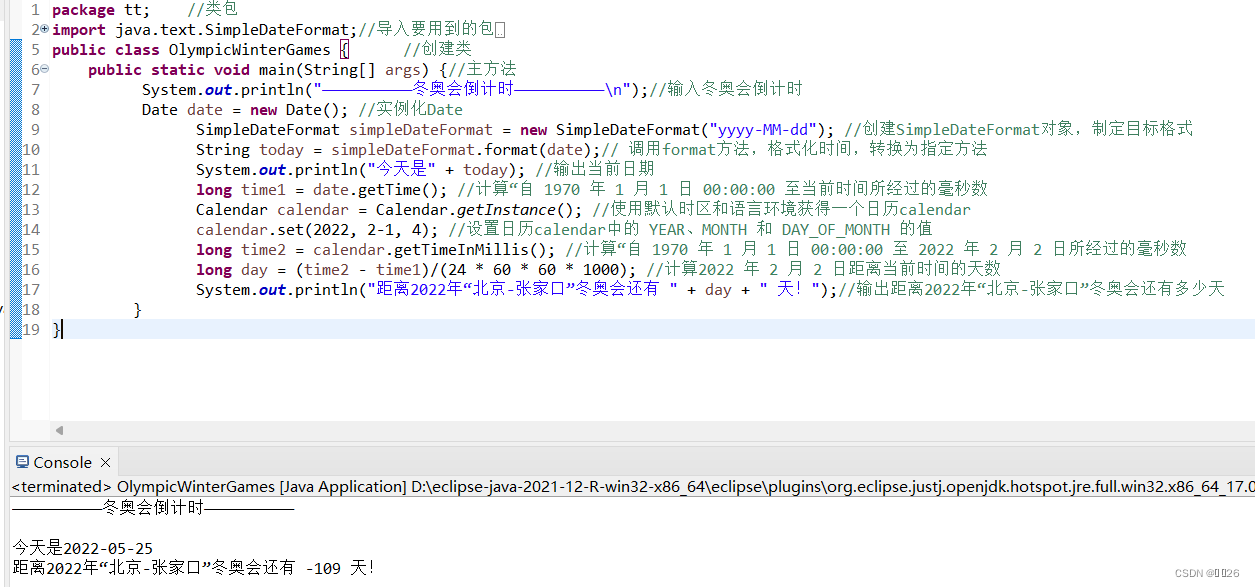# Java中的類，第九章 java常用類

## 9.1 包裝類

Java是一種面向對象的語言，但在Java中不能定義基本數據類型的對象，為了能將基本數據類型視為對象進行處理，Java 提出了包裝類的概念，它主要是將基本數據類型封裝在包裝類中，如int型數值的包裝類Integer, boolean 型的包裝類Boolean等，這樣便可以把這些基本數據類型轉換為對象進行處理。Java 中的包裝類及其對應的基本數據類型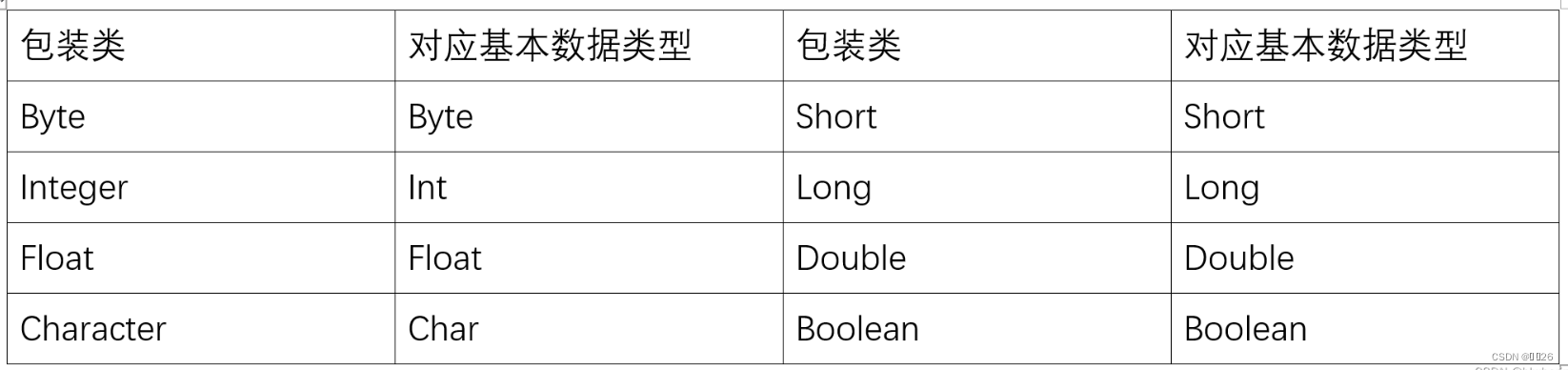Java中的類？

## ?9.1.1 ?integer類

javalang包中的Integer類、Byte 類、Short類和Long類，分別將基本數據類型int. bye. short

Integer類在對象中包裝了一個基本數據類型int的值，該類的對象包含一個int類型的字段，此外，該類提供了多個方法，能在int類型和String類型之間互相轉換，同時還提供了其他一些處理int類型時非常有用的常量和方法。

### 1.構造方法

Integer類有以下兩種構造方法。
(1) Integer ( int number)

Integer number = new Integer(7);

(2) Integer (String str)

Integer number = new Integer("45");

### 2.常用方法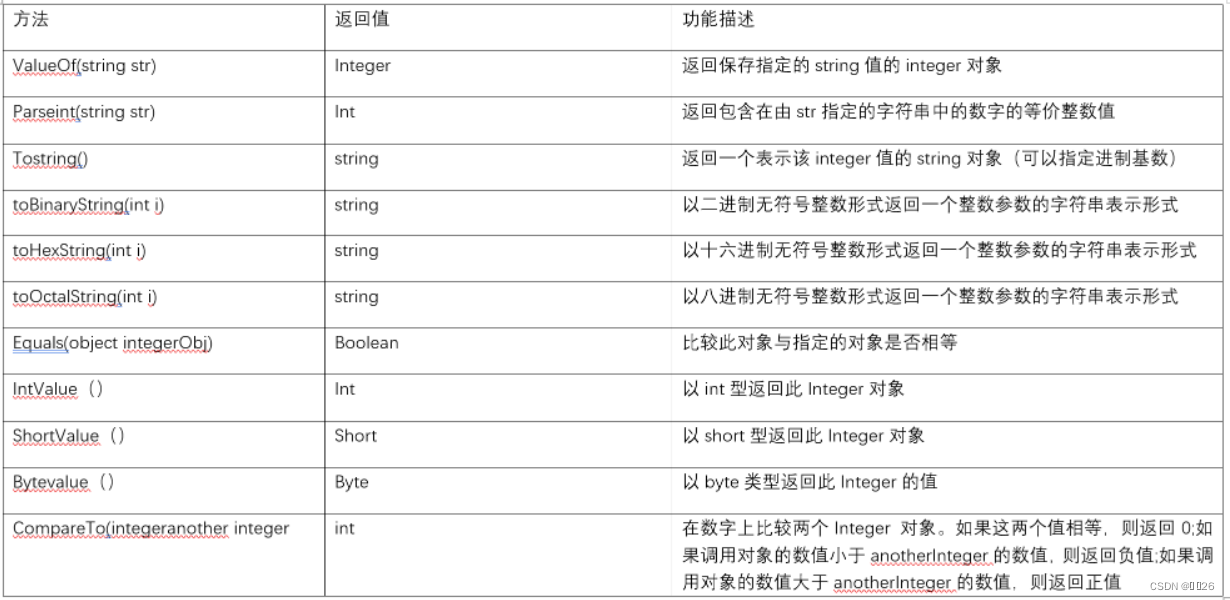?創建一個Demo類，其中首先使用Integer類的parseInt 方法將一個字符串轉換為imt數據;然后創建一個Integer對象，并調用其equals方法與轉換的int數據進行比較;最后演示使用Integer類的toBinaryString方法、toHexString方法、toOctalString 方法和toString方法將int數據轉換為二進制、十六進制、八進制和不常使用的十五進制表示形式。

``````package 第九章;     //類包
public class ss {      //創建類public static void main(String[] args) {   //主方法int num = Integer.parseInt("456");   //將字符串轉換為int類型Integer iNum = Integer.valueOf("456");  //通過構造函數創建一個Integer對象System.out.println("int數據與Integer對象比較:"+iNum.equals(num));   //比較int數據與integer對象比較String str2 = Integer.toBinaryString(num);  //獲取數字的二進制表示String str3 = Integer.toHexString(num);   //獲取數字的十六進制表示String str4 = Integer.toOctalString(num);   //獲取數字的八進制表示String str5 = Integer.toString(num,5);   //獲取數字的十五進制表示System.out.println("456的二進制表示為:"+str2);   //輸出二進制的值System.out.println("456的十六進制表示為:"+str3);   //輸出十六進制的值System.out.println("456的八進制表示為:"+str4);   //輸出八進制的值System.out.println("456的十五進制表示為:"+str5);  //輸出十五進制的值}}``````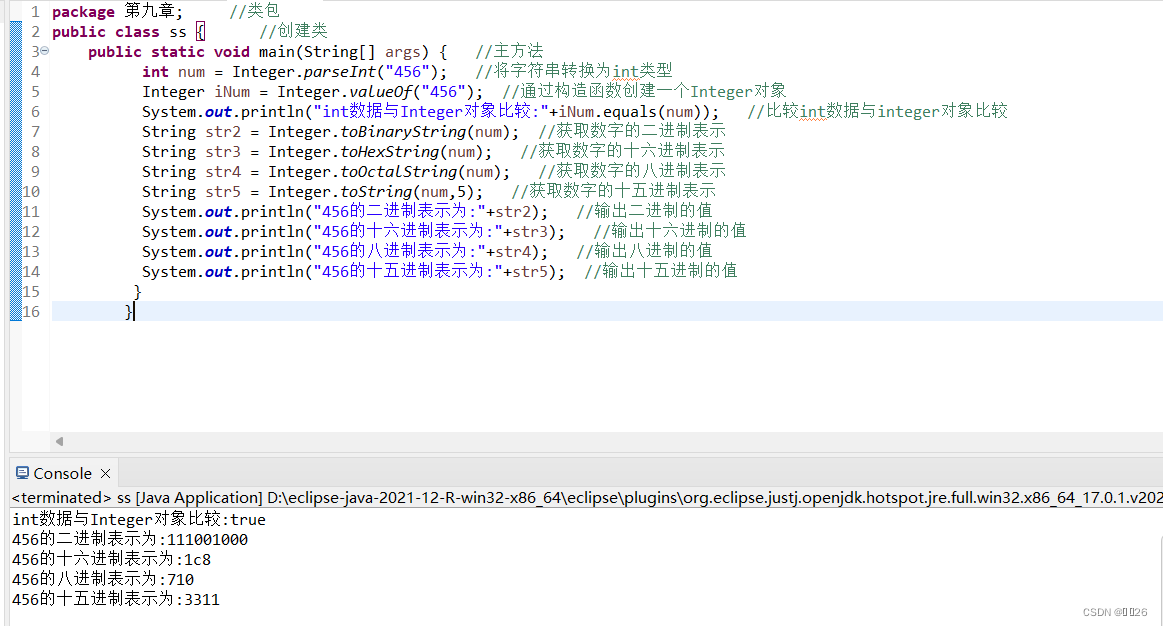### 3.常量

nteger類提供了以下4個常量:

MAX_VALUE:表示int類型可取的最大值，即2平方31-1.

MIN_VALUE:表示int類型可取的最小值，即-2平方31

SIZE:用來以二進制補碼形式表示int值的位數。

TYPE:表示基本類型int的Class實例。

``````package 第九章;    //類包
public class dd {    //創建類public static void main(String[] args) {   //主方法int maxint = Integer.MAX_VALUE;  //獲取Integer類的常量值int minint = Integer.MIN_VALUE;   //獲取Integer類的常量值int intsize = Integer.SIZE;   //獲取Integer類的常量值System.out.println("int類型可取的最大值是:"+maxint);   //將常量值輸出System.out.println("int類型可取的最小值是:"+minint);   //將常量值輸出System.out.println("int類型的二進制位數是:"+intsize);   //將常量值輸出}}``````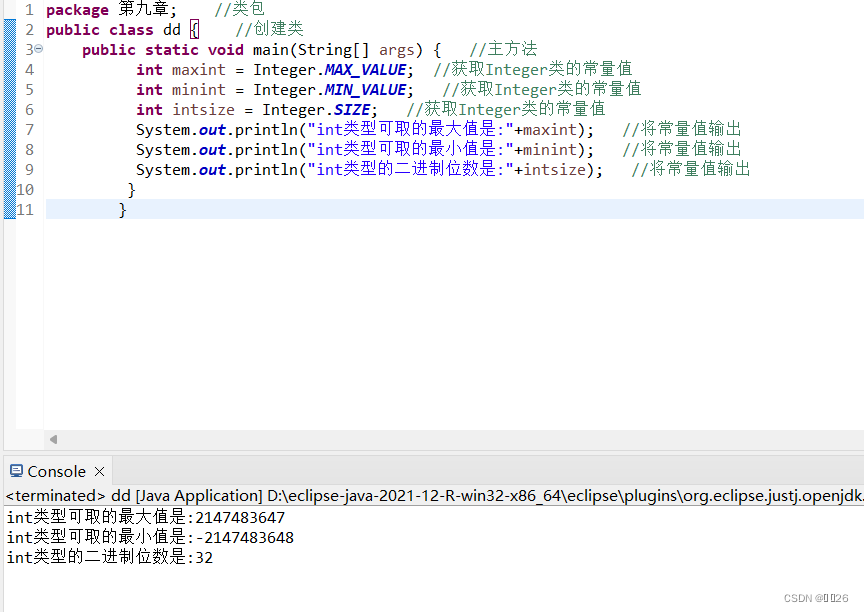## ?9.1.2 ?Double類

Double類和Float類是對double、float 基本類型的封裝，它們都是Number類的子類，都是對小數進行操作，所以常用方法基本相同，本節將對Double類進行介紹。對于Float類可以參考Double類的相關介紹。

Double類在對象中包裝一個基本類型為double的值，每個Double類的對象都包含一個 double類型的字段。此外，該類還提供多個方法，可以將double轉換為String,將String轉換為double,也提供了其他一些處理 double時有用的常量和方法。

### 1.構造方法

Double類提供了以下兩種構造方法來獲得Double類對象。
(1 ) Double(double value)

Double number = new Double(3.14);

(2 ) Double(String str)

Doble number = new Double (“3.14”);

### 2.常用方法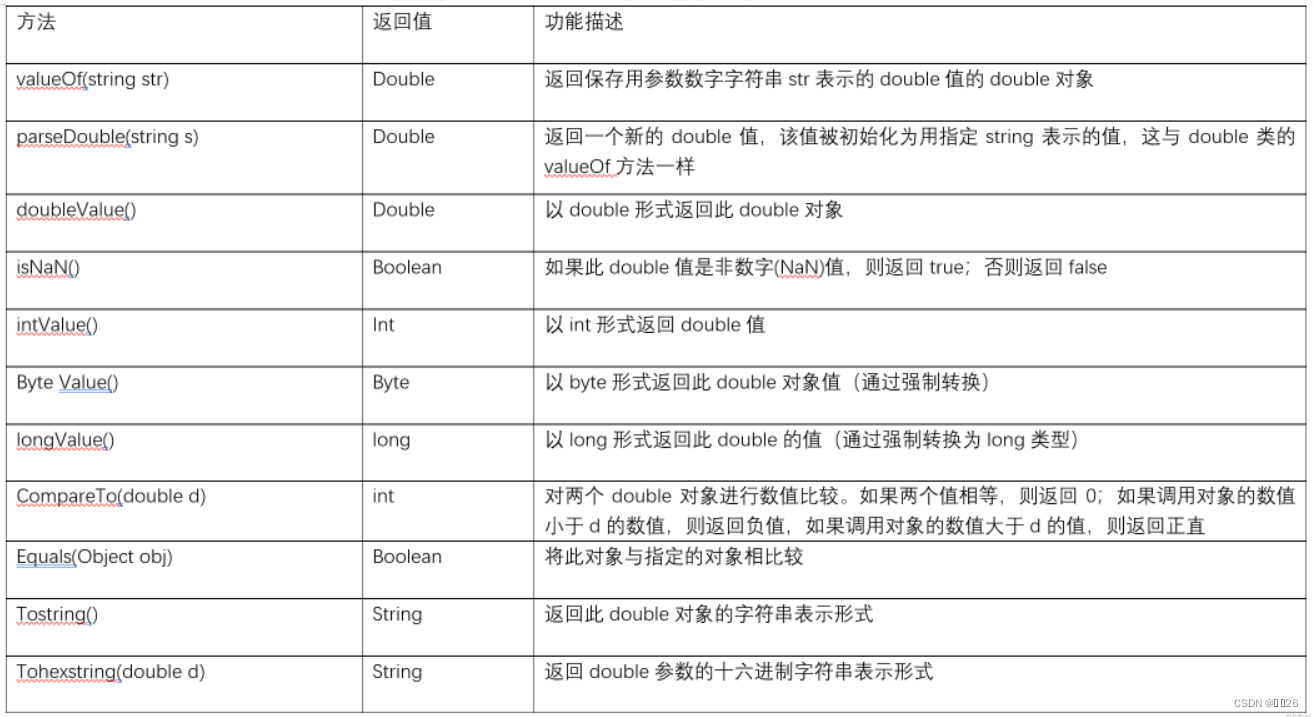?下面通過一個實例演示double類的常用方法的使用

``````package 第九章;    //類包
public class dd {  //創建類public static void main(String[] args) {   //主方法Double dNum = Double.valueOf("3.14");   //通過構造函數創建一個Double對象System.out.println("3.14是否為非數字值:"+ Double.isNaN(dNum.doubleValue()));   //判斷是否為非數字值System.out.println("3.14轉換為int值為:"+ dNum.intValue());  //轉換為int類型System.out.println("值為3.14的Double對象與3.14的比較結果:"+dNum.equals(3.14));  //判斷大小System.out.println("3.14的十六進制表示為:"+Double.toHexString(dNum));  //轉換為十六進制}}``````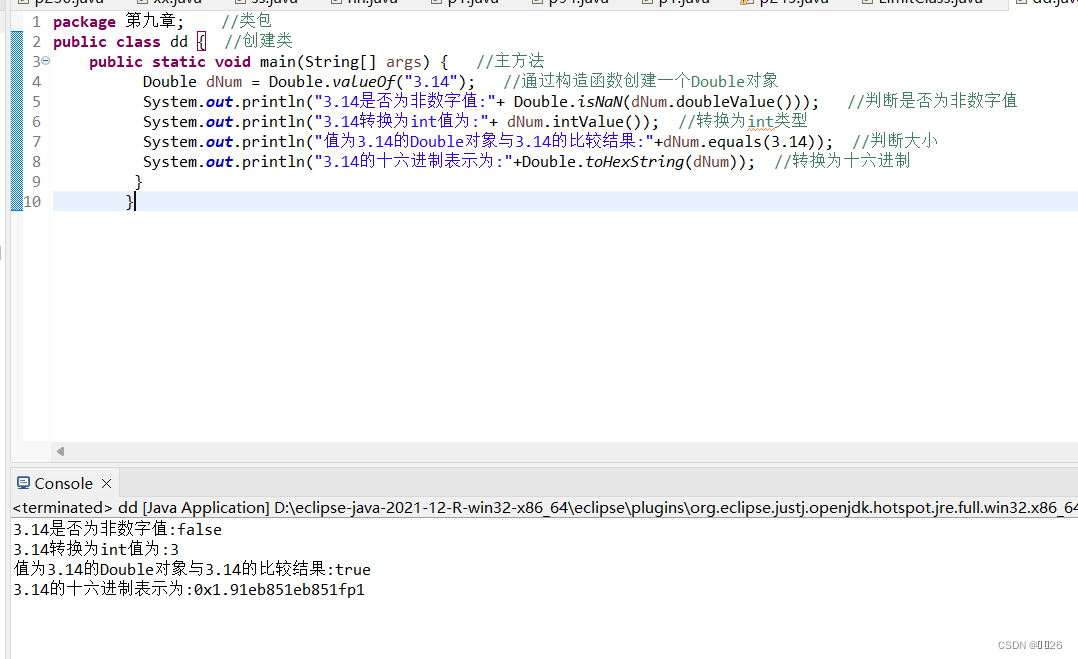### 3.常量

Double類主要提供了以下常量：

?(1) MAX EXPONENT:返回int值，表示有限double變量可能具有的最大指數。
(2) MIN EXPONENT:返回int值，表示標準化double變量可能具有的最小指數。
(3) NEGATIVE INFINITY:返回double值，表示保存double 類型的負無窮大值的常量，
(4) POSITIVE INFINITY:返回double值，表示保存double類型的正無窮大值的常量.

## 9.1.3 Boolean類

Boolean類將基本類型為boolean的值包裝在一個對象中。一個Boolean,類型的對象只包含一個類型為bolean的字段。此外，此類還為boolan和String的相互轉換提供了許多多方法，并提供了處理boolean時非常有用的其他一些常量和方法。

### 1.構造方法

Boolean類提供了以下兩種構造方法來獲得Boolean類對象。
(1) Boolean(boolean value)

Boolean b = new Boolean (true) ;

(2) Boolean(String str)

Boolean bool = new Boolean("ok");

### 2.常用方法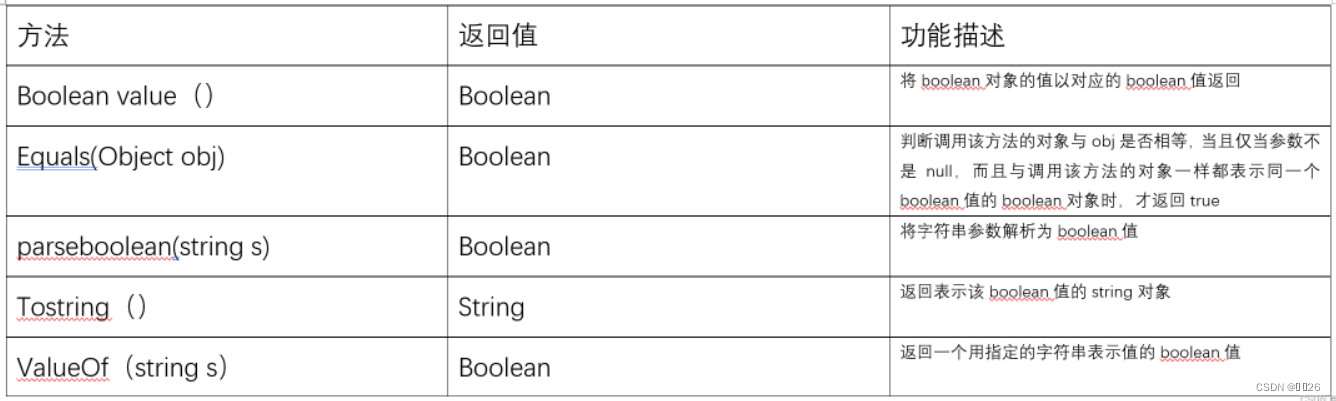``````package 第九章;   //類包
public class dd {   //創建類public static void main(String[] args) {   //主方法Boolean b1 = new Boolean(true);   //創建Boolean1對象Boolean b2 = new Boolean("ok");   //創建Boolean2對象System.out.println("b1:"+b1.booleanValue());   //輸出Boolean1對象結果System.out.println("b2:"+b2.booleanValue());   //輸出Boolean2對象結果}
}``````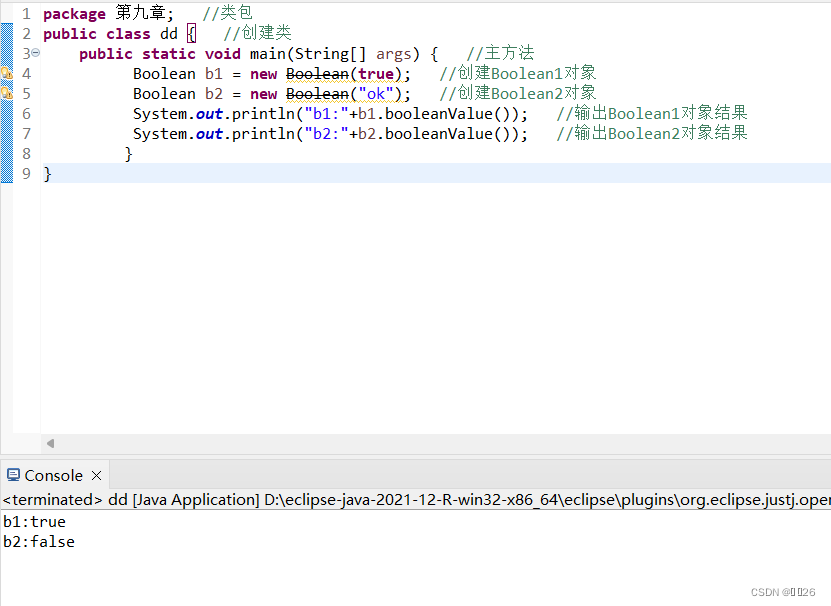3.常量

Boolean提供了以下3個常量:

(1) TRUE: 對應基值true的Boolean對象。
(2) FALSE: 對應基值false的Boolean對象。
(3)TYPE:基本類型boolean的Class對象。

## 9.1.4 ?Character類

Cancer類在對象中包裝一個基本類型為 chara的值，該類提供了多種方法，以確定字符的類期(小寫字母、數字等)，并可以很方便地將字符從大寫轉換成小寫，反之亦然。

### 1.構造方法

Character類的構造方法語法如下:

Character (char value)

Character mychar = new Character('s');

### 2.常用方法

Characer類提供了很多方法來完成對字符的操作，常用的方法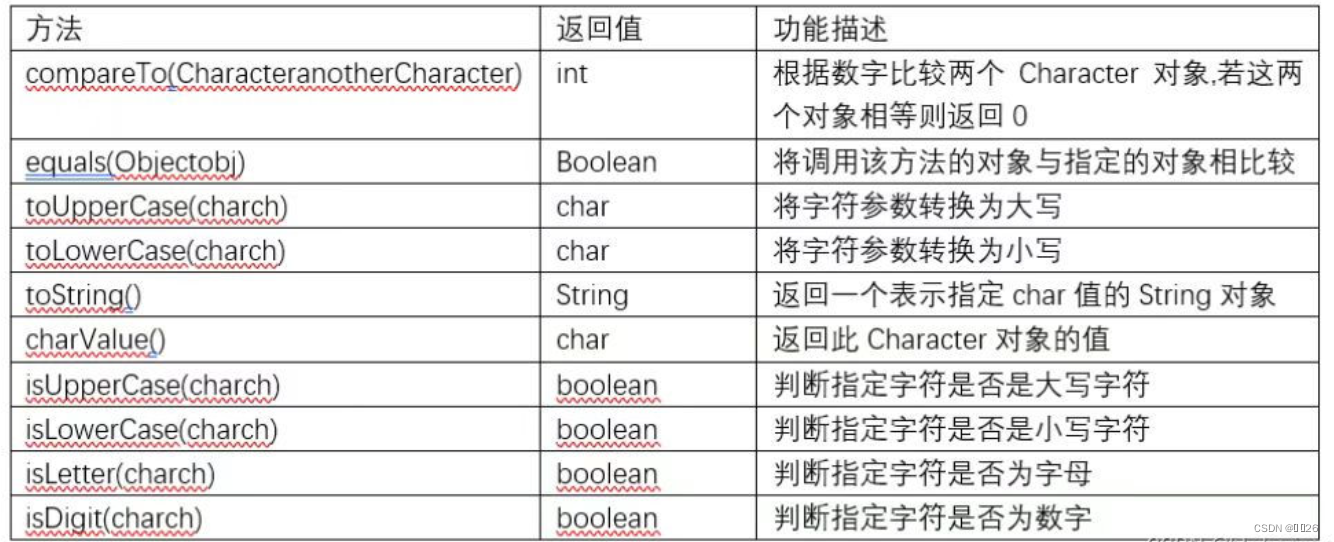``````package 第九章;    //類包
public class dd {   //創建類public static void main(String[] args) {   //主方法Character mychar1 = new Character('A');  //聲明Character對象Character mychar2 = new Character('a');   //聲明Character對象if (Character.isUpperCase(mychar1)) {  //判斷是否為大寫字母System.out.println(mychar1+"是大寫字母");   //為大寫字母System.out.println("轉換為小寫字母的結果:"+Character.toLowerCase(mychar1));   //轉換為小寫字母}if(Character.isLowerCase(mychar2)) {    //判斷是否為小寫字母System.out.println(mychar1+"是小寫字母");  //為小寫字母System.out.println("轉換為小寫字母的結果:"+Character.toUpperCase(mychar1));   //轉換為大寫字母}}
}``````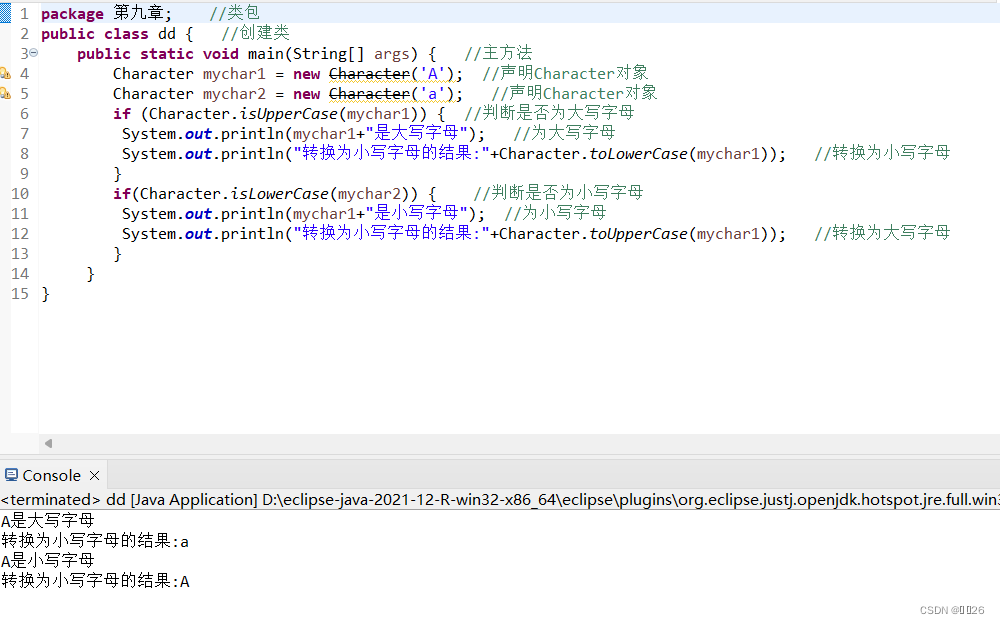### 3.常量

Character類提供了大量表示特定字符的常量，例如:

( 1 ) CONNECTOR PUNCTUATION: 返回byte型值，表示Unicode規范中的常規類別“Pc”。
(2) UNASSIGNED:返回byte型值，表示Unicode規范中的常規類別“Cn”。
(3) TITLECASE LETTER:返回byte型值，表示Unicode規范中的常規類別“Lt”。

## 9.1.5 ?Number類

Number類的方法分別被Number的各子類所實現，也就是說，在Number類的所有子類中都包含以上這幾種方法。

## 9.2.1 ?Math類概述

Math類表示數學類，它位于java. ang包中，由系統默認調用，該類中提供了眾多數學函數方法，主要包括三角函數方法，指數函數方法，取整函數方法，取最大值、最小值以及絕對值函數方法，這些方法都被定義為static 形式，因此在程序中可以直接通過類名進行調用。使用形式如下:

Math.數學方法

Math. PI ? ? ? ?//表示圓周率PI的值

Math.E ? ? ? ? //表示自然對數底數e的值

System. out.println("圓周率π的值為:" + Math.PI);

System. out .println("自然對數底數e的值為:”+ Math.E);

### 9.2.2 ?常用屬性運算方法

Math類中的常用數學運算方法較多，大致可以將其分為4大類別，分別為三角函數方法，指數函數方法，取整函數方法以及取最大值、最小值和絕對值函數方法，下面分別進行介紹。

### 1.三角函數方法

Math類中包含的三角函數方法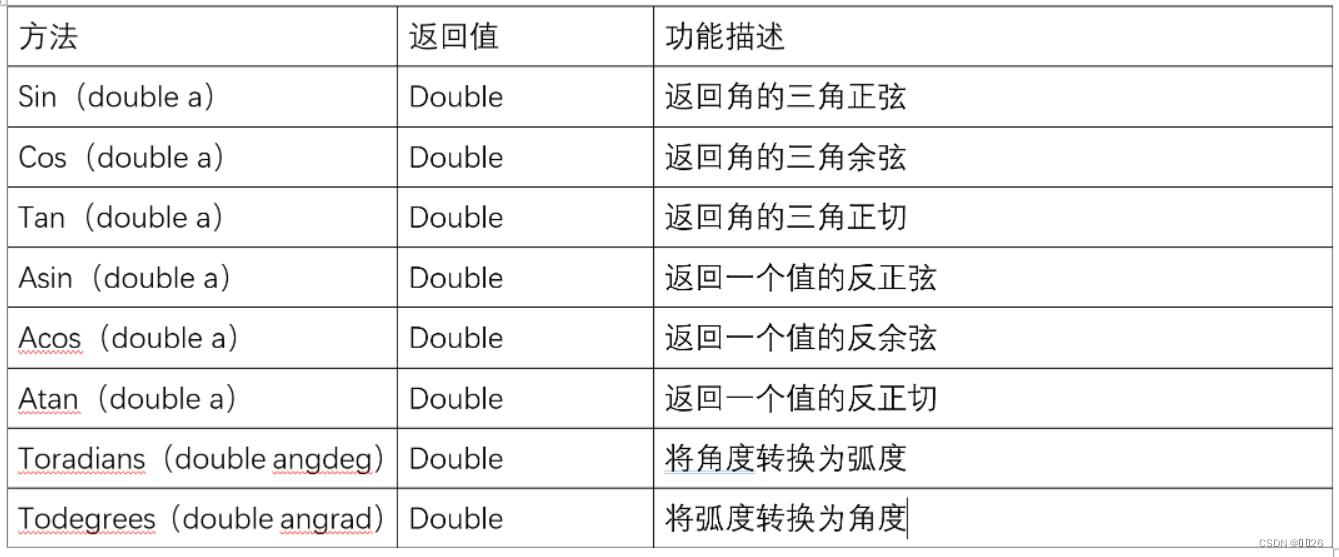``````package 第九章;    //類包
public class dd {    //創建類  public static void main(String[] args) {//主方法System.out.println("90度的正弦值:"+Math.sin(Math.PI/2));//取90°的正弦System.out.println("0度的余弦值:"+Math.cos(0));//取0°的余弦System.out.println("60度的正切值:"+Math.tan(Math.PI/3));//取60°的正切System.out.println("2的平方根與2商的反弦值："+ Math.asin(Math.sqrt(2) / 2));// 取2的平方根與2商的反弦System.out.println("2的平方根與2商的反余弦值："+ Math.acos(Math.sqrt(2) / 2));// 取2的平方根與2商的反余弦System.out.println("1的反正切值：" + Math.atan(1)); // 取1的反正切System.out.println("120度的弧度值：" + Math.toRadians(120.0));// 取120度的弧度值System.out.println("π/2的角度值：" + Math.toDegrees(Math.PI / 2));// 取π/2的角度}}``````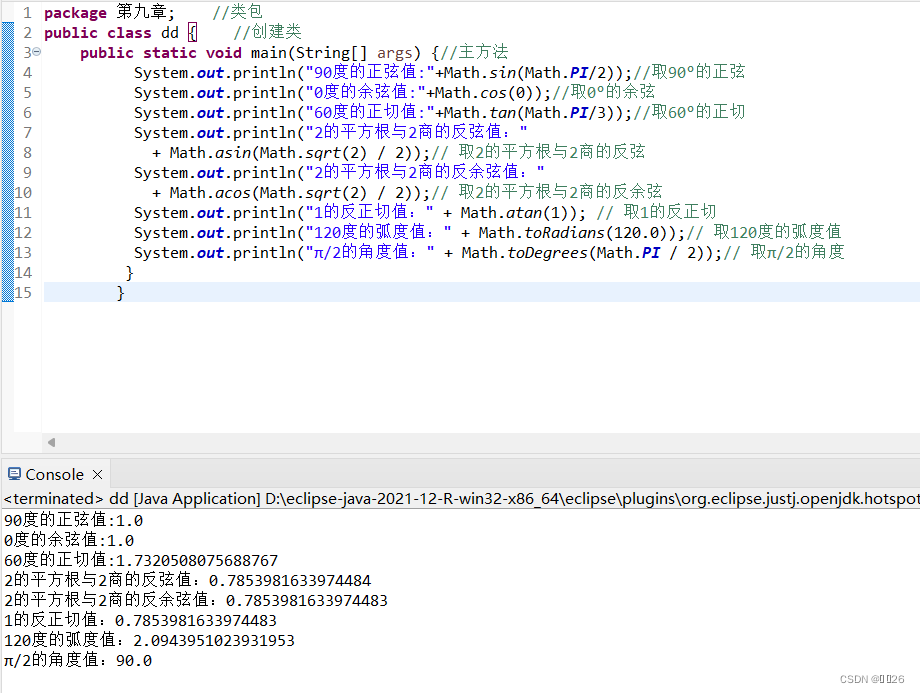### 2.指數函數方法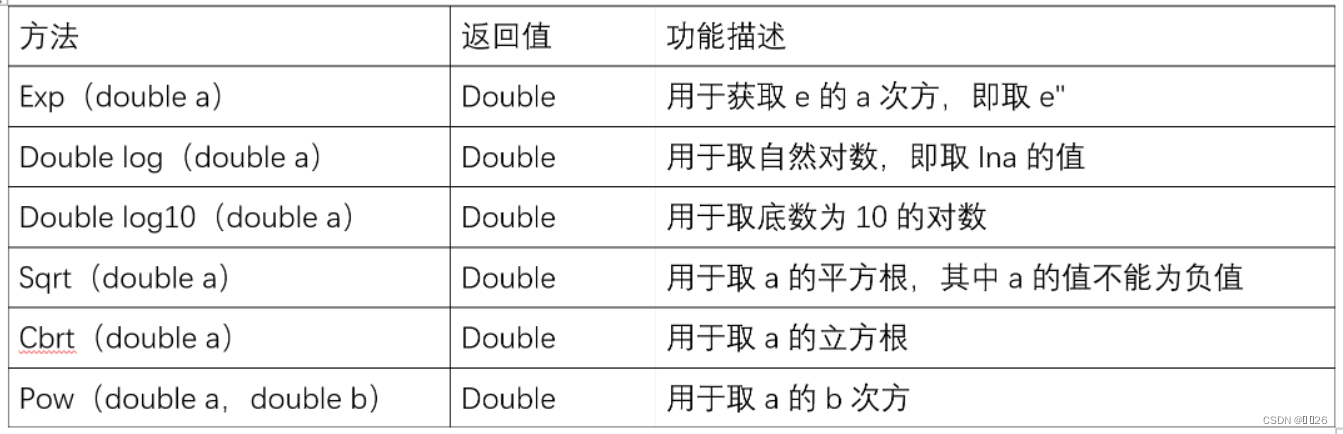``````package 第九章;   //類包
public class dd {    //創建類public static void main(String[] args) {//主方法System.out.println("e的平方值:"+Math.exp(2));//取e的2次方System.out.println("以e為底2的對數值:"+Math.log(2));//取以e為底2的對數System.out.println("以10為底2的對數值:"+Math.log10(2));//取以10為底2的對數System.out.println("4的平方根值:"+Math.sqrt(4));//取4的平方根System.out.println("8的立方根值:"+Math.cbrt(8));//取8的立方根System.out.println("2的2次方值:"+Math.pow(2,2));//取2的2次方}}``````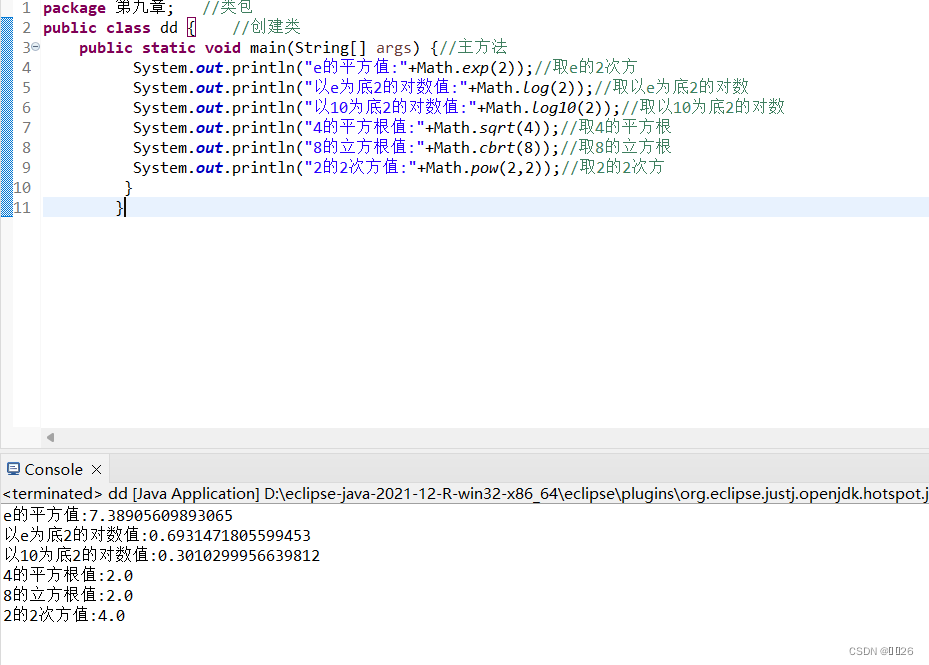### 3.取整函數方法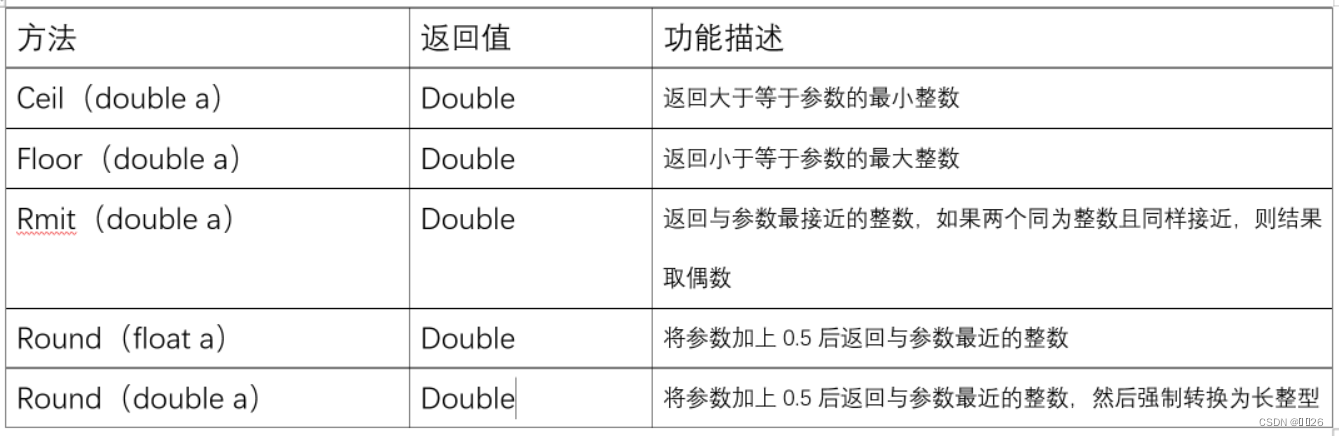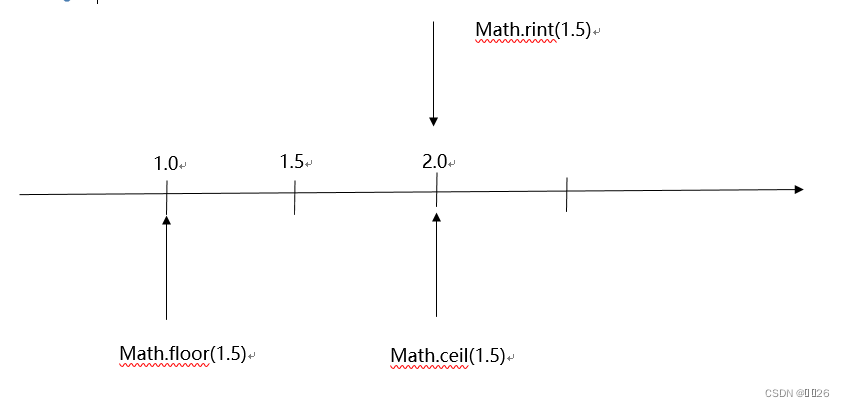``````package tt;       //類包
public class IntFunction {   //創建類public static void main(String[] args) {//主方法System.out.println("使用ceil()方法取整:"+Math.ceil(5.2));//返回第一個大于參數的整數System.out.println("使用floor()方法取整"+Math.floor(2.5));//返回第一個小于等于參數的整數System.out.println("使用rint()方法取整"+Math.rint(2.7));//返回與參數最接近的整數System.out.println("使用rint()方法取整"+Math.rint(2.5));//返回與參數最接近的整數System.out.println("使用round()方法取整"+Math.round(3.4f));//將參數加上0.5后返回最接近的整數System.out.println("使用round()方法取整"+Math.round(2.5));//將參數加上0.5后返回最接近的整數,并將結果強制轉換為長整型}}``````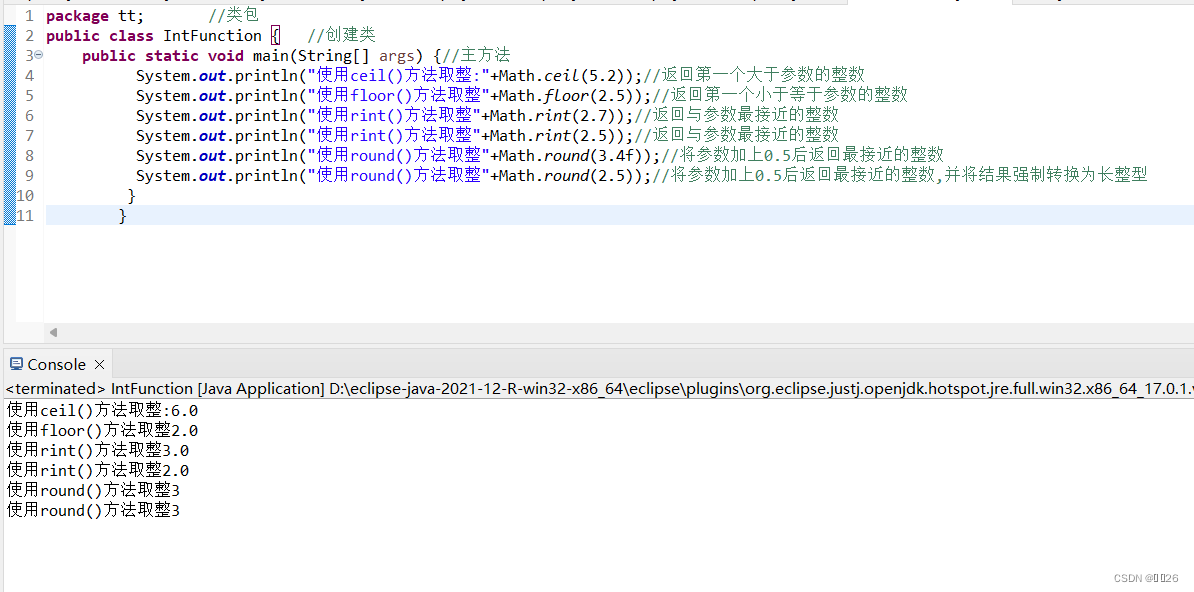### ?4.取最大值、最小值、絕對值函數方法

Math類還有一些常用的數據操作方法，比如取最大值、最小值、絕對值等，它們的說明如表

``````package tt;      //類包
public class AnyFunction {       //創建類public static void main(String[] args) {//主方法System.out.println("4和8較大者:"+Math.max(4,8));//取兩個參數的最小值System.out.println("4.4和4較大者:"+Math.min(4.4,4));//取兩個參數的最大值System.out.println("-7的絕對值:"+Math.abs(-7));//取參數的絕對值}}``````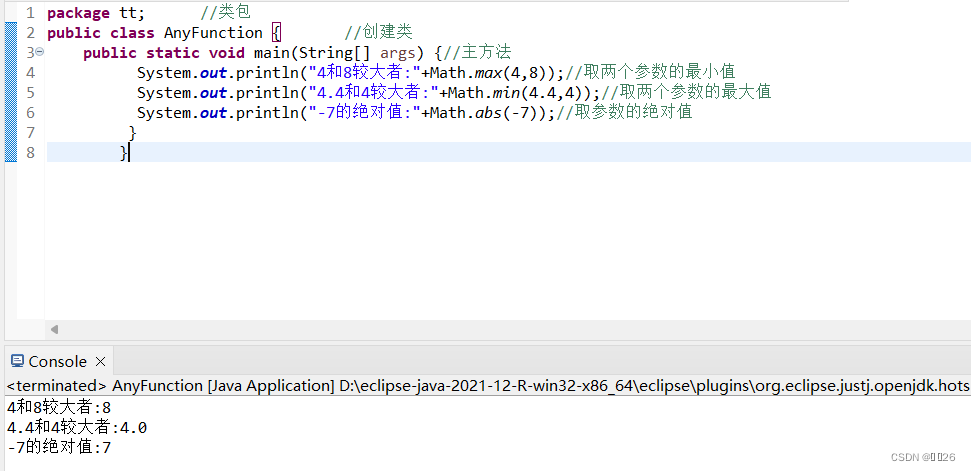## 9.3 ?隨機數?

### 9.3.1 Math.random()方法

``````package tt;    //類包
import java.util.Scanner;//導入要用到的包
public class NumGame {     //創建類public static void main(String[] args) {//主方法System.out.println("——————猜數字游戲——————\n");//輸出猜數字游戲int iNum;//定義iNumint iGuess;//定義iGuessScanner in = new Scanner(System.in);// 創建掃描器對象，用于輸入iNum = (int) (Math.random() * 100);// 生成1到100之間的隨機數System.out.print("請輸入你猜的數字：");//輸入猜的數字iGuess = in.nextInt(); // 輸入首次猜測的數字while ((iGuess != -1) && (iGuess != iNum))// 判斷輸入的數字不是-1或者基準數{if (iGuess < iNum)// 若猜測的數字小于基準數，則提示用戶輸入的數太小，并讓用戶重新輸入{System.out.print("太小，請重新輸入：");   //請重新輸入iGuess = in.nextInt();     //輸出} else// 若猜測的數字大于基準數，則提示用戶輸入的數太大，并讓用戶重新輸入{System.out.print("太大，請重新輸入：");     //請重新輸入iGuess = in.nextInt();     //輸出}}if (iGuess == -1)// 若最后一次輸入的數字是-1，循環結束的原因是用戶選擇退出游戲{System.out.println("退出游戲！");    //退出游戲} else// 若最后一次輸入的數字不是-1，用戶猜對數字，獲得成功，游戲結束{System.out.println("恭喜你，你贏了，猜中的數字是：" + iNum);   //輸出猜中的數字}System.out.println("\n———————游戲結束———————");//輸出游戲結束}}``````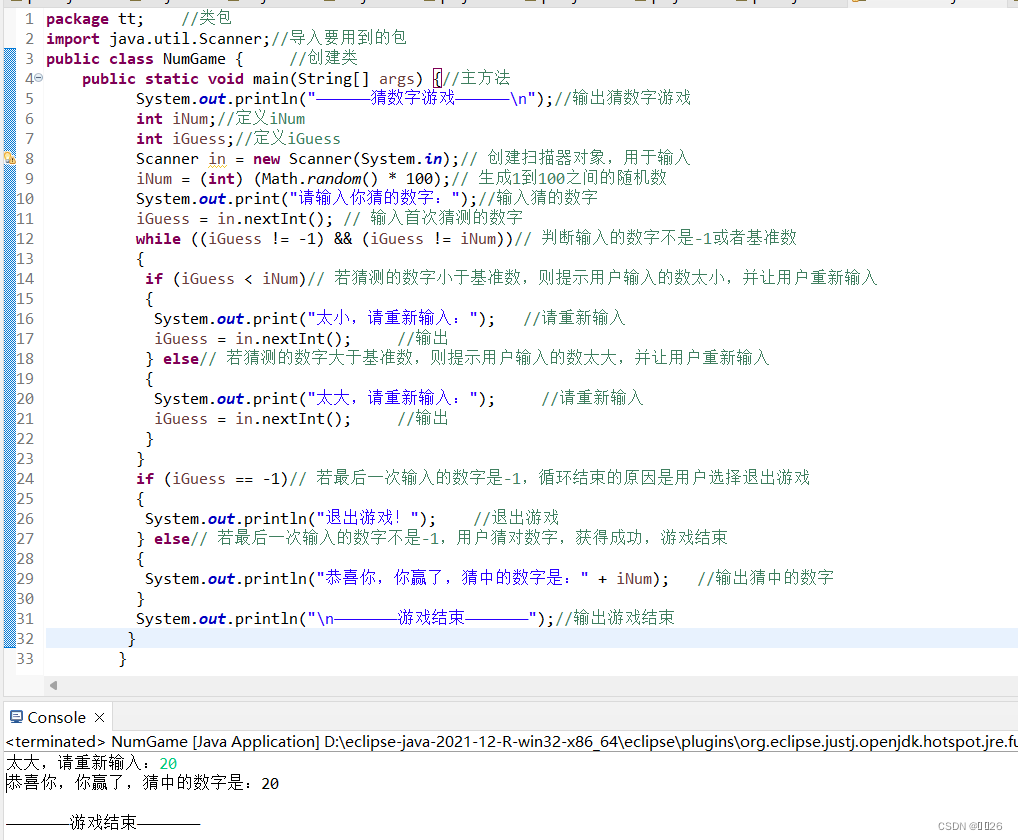(char) ('a'+Math. random()*('z'-'a'+1)) ;

(char) (chal +Math. random() * (cha2-cha1+1)) ;

``````package tt;      //類包
public class MathRandomChar {    //創建類public static char GetRandomChar(char cha1, char cha2) {//定義獲取任意字符之間的隨機字符return (char) (cha1 + Math.random() * (cha2 - cha1 + 1));//參數設置}public static void main(String[] args) {//主方法System.out.println("任意小寫字符" + GetRandomChar('a', 'z'));// 獲取a~z之間的隨機字符System.out.println("任意大寫字符" + GetRandomChar('A', 'Z'));// 獲取A~Z之間的隨機字符System.out.println("0到9任意數字字符" + GetRandomChar('0', '9'));// 獲取0~9之間的隨機字符}}``````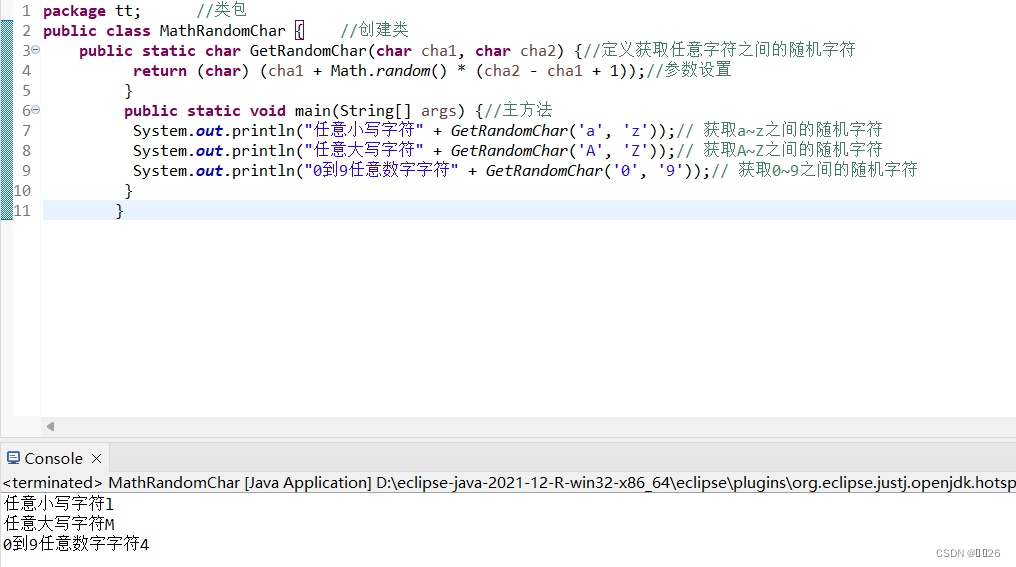## 9.3.2 ?Randon類

Random r=new Random() ;

Random r=newRandom (seedValue);

r: Random類對象。

seedValue:: 隨機數生成器的種子。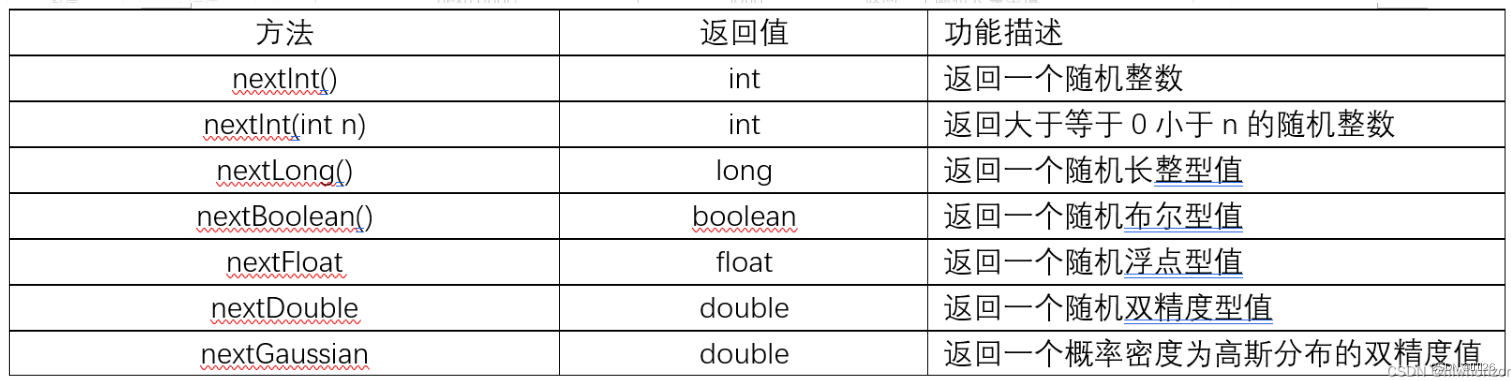``````package tt;   //類包
import java.util.Random;//導入要用到的包
import java.util.Scanner;//導入要用到的包
public class RedBags {    //創建類public static void main(String[] args) {//主方法System.out.println("————模擬微信搶紅包————"); //輸入————模擬微信搶紅包————Scanner sc = new Scanner(System.in);//控制臺輸入System.out.print("請輸入要裝入紅包的總金額(元):");double total=sc.nextDouble();//輸入“紅包的總金額” System.out.print("請輸入紅包的個數(個):");//輸入紅包個數int bagsnum=sc.nextInt();//輸入“紅包的個數”double min=0.01;//初始化“紅包的最小金額”Random random =new Random();//創建隨機數對象random for (int i=1;i<bagsnum;i++){//設置“循環”/**通過公式模擬數學中的離散模型計算一個紅包可以放的最大金額*本次紅包可用最大金額=可分配金額-(紅包總數-已發出的紅包數)*紅包的最小金額*/double max=total-(bagsnum-i)*min;double bound=max-min;// 設置隨機金額的取值范圍 /** 據隨機金額的取值范圍，隨機生成紅包金額。* 由于nextInt(int bound)只能用整型做參數，所以先將bound乘100(小數點向右挪兩位*獲取到一個整數后，將這個整數除100(小數點向左挪兩位)并轉換成與金額相同的浮點類型*/double safe=(double)random.nextInt((int)(bound*100))/100;double money=safe+min;//最后加上紅包的最小金額，以防safe出現0值total=total- money;//替換total的值System.out.println("第" + i + "個紅包:" + String.format("%.2f",money) + "元");//輸出結果}System.out.println("第" + bagsnum + "個紅包:" + String.format("%.2f", total)+ "元");//輸出結果sc.close();// 關閉控制臺輸入}
}``````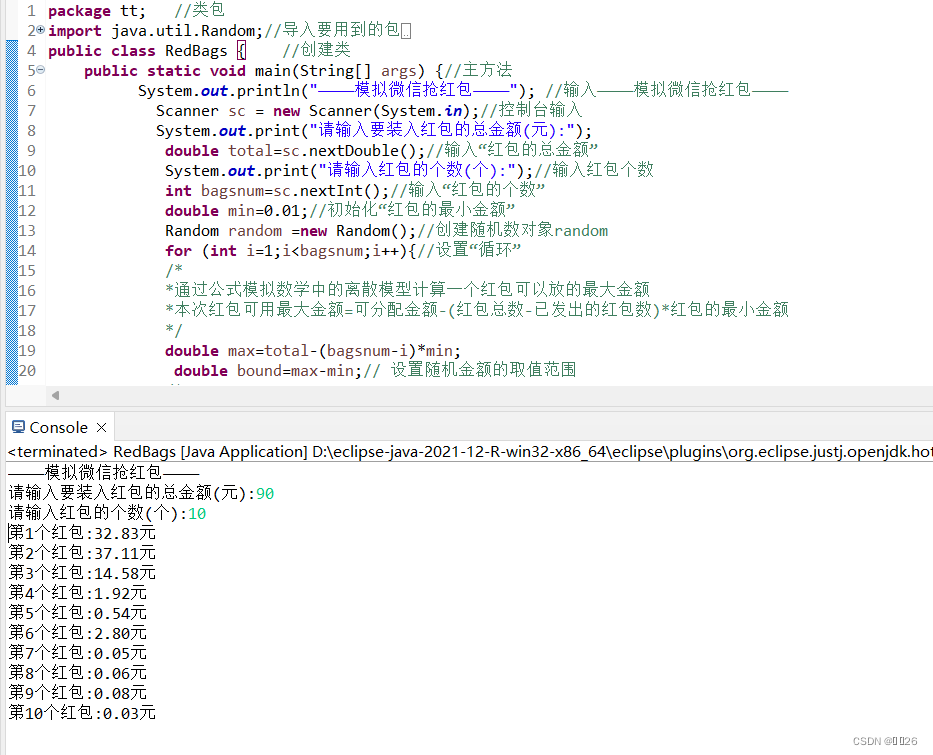### 9.4.1 ?Date類

Date類用于表示日期時間，它位于java.util包中，程序中使用該類表示時間時，需要使用其構造方法創建Date類的對象，其構造方法及說明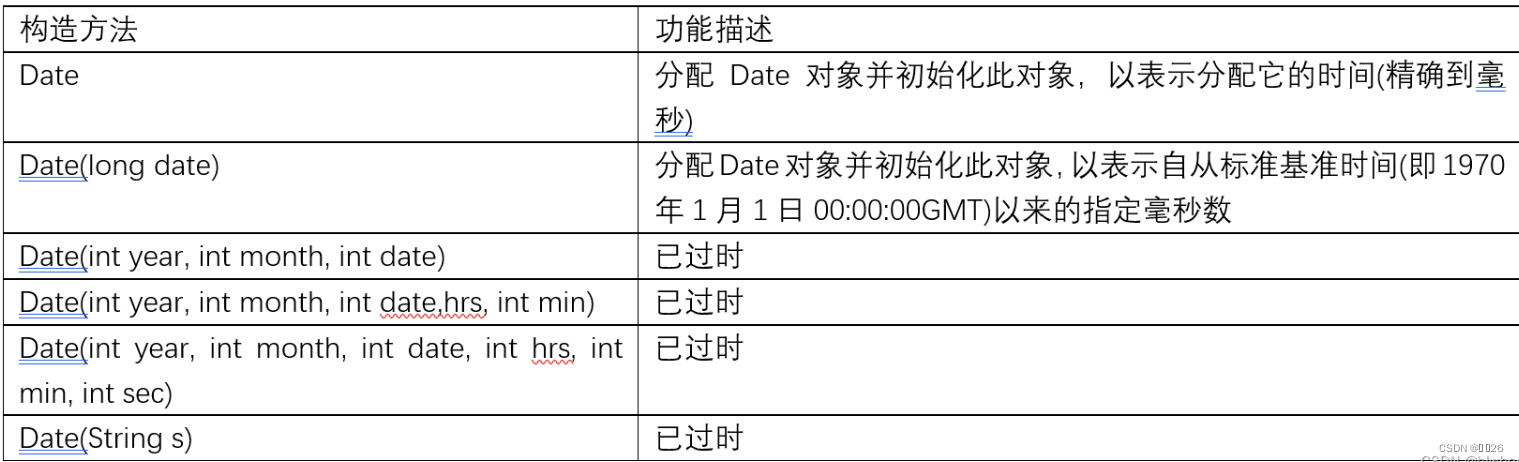long timeMillis = System. currentTimeMillis() ;
Date date=new Date (timeMillis) ;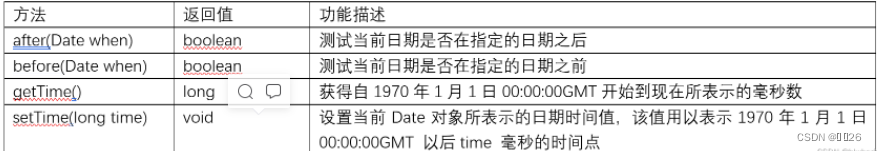``````package tt;    //類包
import java.util.Date;//導入用到的包
public class DateTest {   //創建類public static void main(String[] args) {//主方法Date date = new Date();     // 創建現在的日期long value = date.getTime();    // 獲得毫秒數System.out.println("日期：" + date);//輸入日期+dateSystem.out.println("到現在所經歷的毫秒數為： " + value);//輸出毫秒數}}``````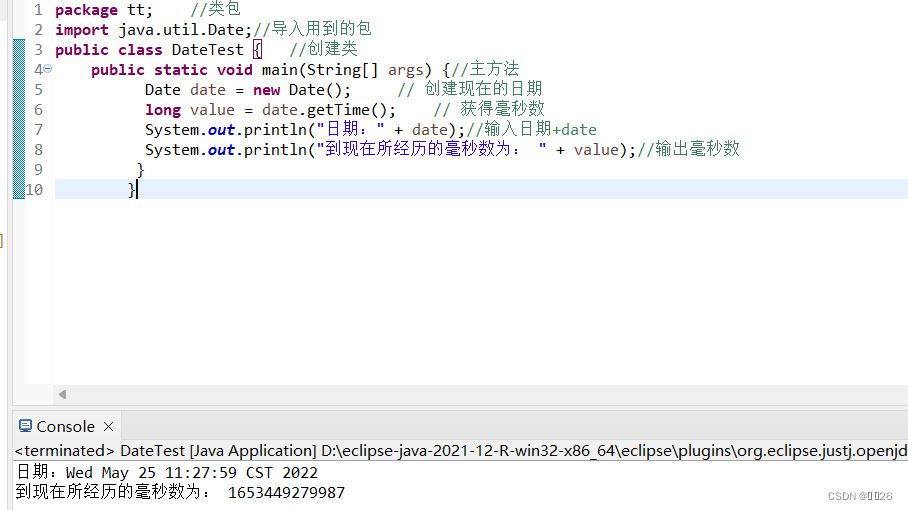DateFomat類是日期/時間格式化子類的抽象類，它位于java.tet 包中，可以按照指定的格式對日期或時間進行格式化。DateFomat 提供了很多類方法，以獲得基于默認或給定語言環境和多種格式化風格的默認日期時間Fomaterer格式化風格包括SHORT、MEDIUM、LONG和FULlL等4種，分別如下:

SHORT: 完全為數字，如12.13.52或3:30pm。

MEDIUM: 較長，如Jan 12, 1952。

LONG: 更長，如January 12, 1952或3:30:32pm。

FULL: 完全指定，如Tuesday、 April 12、 1952 AD或3:30:42pm PST.

DateFormat df = DateFormat .getDateInstance() ;

DateFormat類的常用方法及說明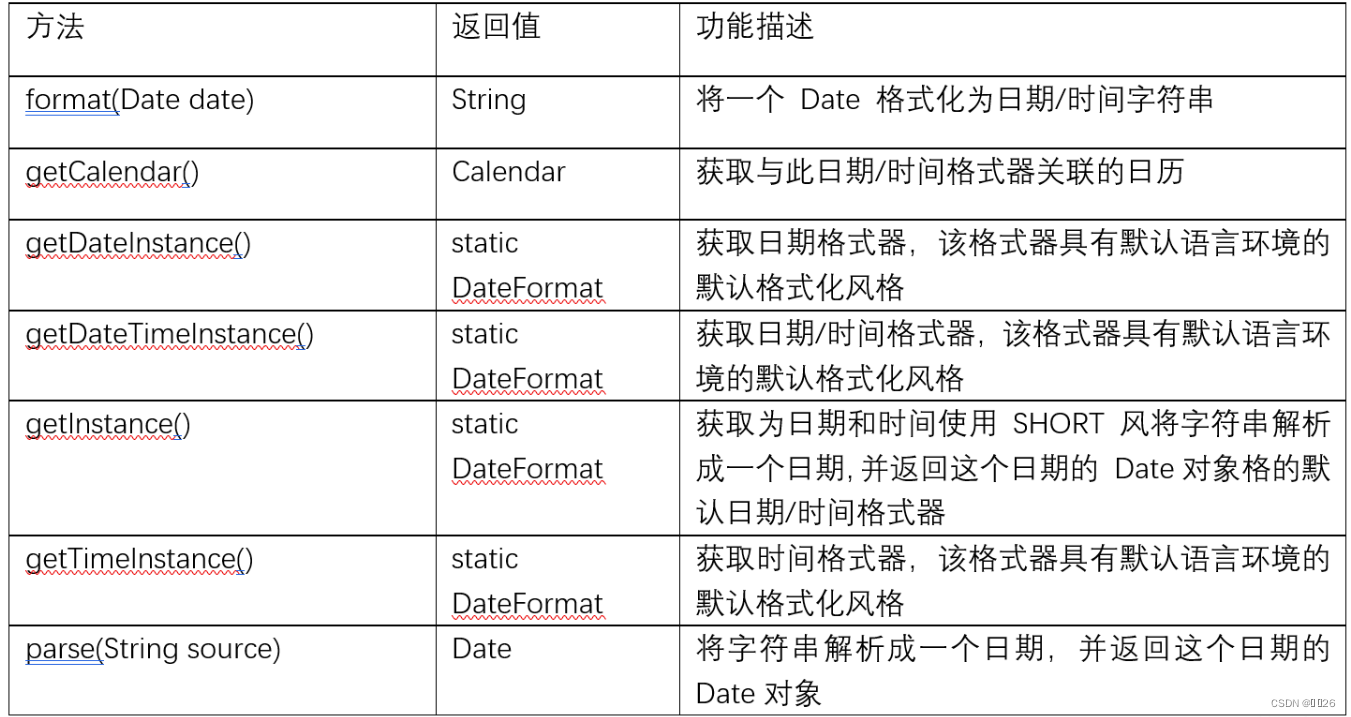由于DateFormat類是一個抽象類， 不能用new創建實例對象，因此，除了使用getXXXInstance方法創建其對象外，還可以使用其子類，例如SimpleDateFormat類，該類是一個以與語言環境相關的方式來格式化和分析日期的具體類，它允許進行格式化(日期->文本)、分析(文本->日期)和規范化。

``````package tt;    //類包
import java.text.DateFormat;//導入用到的包
import java.text.SimpleDateFormat;//導入用到的包
import java.util.Date;//導入用到的包
import java.util.Locale;//導入用到的包
public class DateFormatTest {       //創建類public static void main(String[] args) {//主方法Date date = new Date();// 創建日期DateFormat df1 = DateFormat.getInstance();// 創建不同的日期格式DateFormat df2 = new SimpleDateFormat("yyyy-MM-dd hh:mm:ss EE");//創建yyyy-MM-dd hh:mm:ss EE格式DateFormat df3 = new SimpleDateFormat("yyyy年MM月dd日 hh時mm分ss秒 EE", Locale.CHINA);//創建yyyy年MM月dd日 hh時mm分ss秒 EE", Locale.CHINA格式DateFormat df4 = new SimpleDateFormat("yyyy-MM-dd hh:mm:ss EE", Locale.US);//創建yyyy-MM-dd hh:mm:ss EE", Locale.US格式DateFormat df5 = new SimpleDateFormat("yyyy-MM-dd");//創建yyyy-MM-dd格式DateFormat df6 = new SimpleDateFormat("yyyy年MM月dd日");//創建yyyy年MM月dd日格式System.out.println("-------將日期時間按照不同格式進行輸出------");// 將日期按照不同格式進行輸出System.out.println("按照Java默認的日期格式: " + df1.format(date));//輸出Java默認的日期格式System.out.println("按照指定格式 yyyy-MM-dd hh:mm:ss，系統默認區域:" + df2.format(date));//輸出yyyy-MM-dd hh:mm:ss格式System.out.println("按照指定格式 yyyy年MM月dd日 hh時mm分ss秒，區域為中文 : " + df3.format(date));//輸出yyyy年MM月dd日 hh時mm分ss秒格式System.out.println("按照指定格式 yyyy-MM-dd hh:mm:ss，區域為美國: " + df4.format(date));//輸出yyyy-MM-dd hh:mm:ss格式System.out.println("按照指定格式 yyyy-MM-dd: " + df5.format(date));//輸出yyyy-MM-dd 格式System.out.println("按照指定格式 yyyy年MM月dd日: " + df6.format(date));//輸出yyyy年MM月dd日 格式}
}``````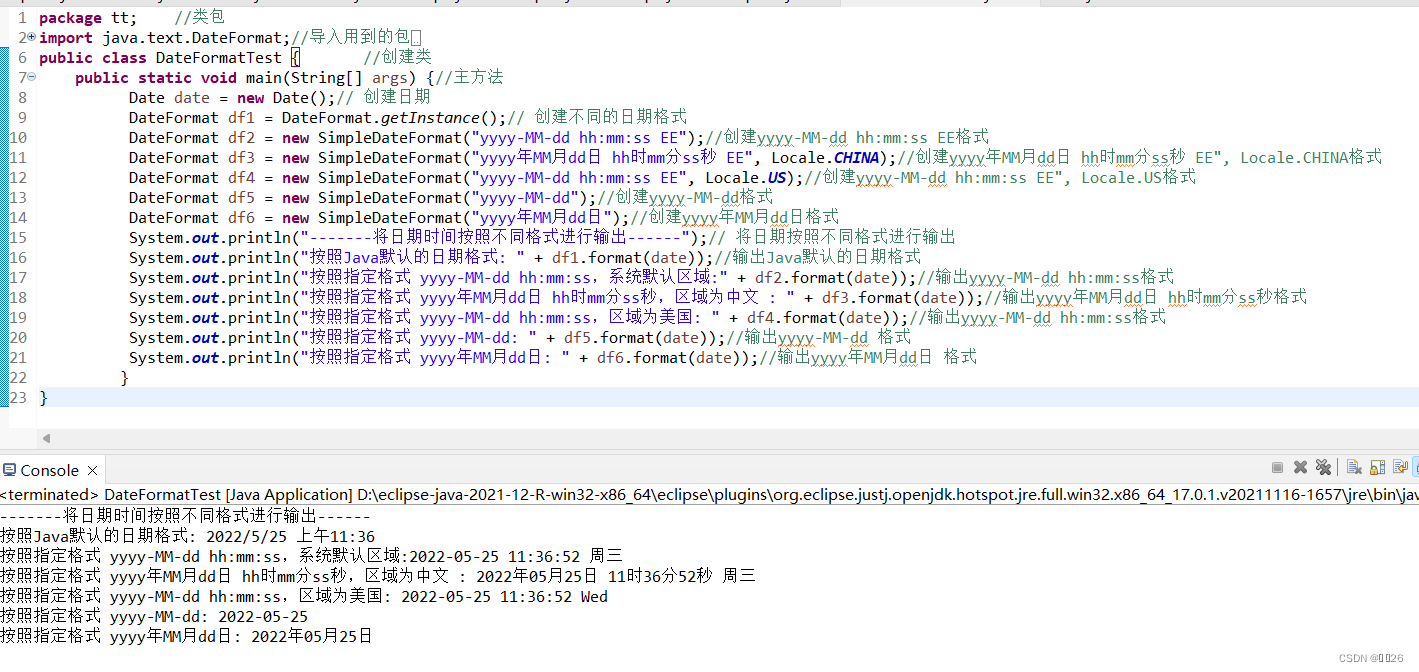### 9.4.2 ?Calendar類

Calendar類是一個抽象類， 它為特 定瞬間與一組諸如YEAR、MONTH、DAY _OF MONTH、HOUR等日歷字段之間的轉換提供了一.些方法，并為操作日歷字段(例如獲得下星期的日期)提供了一些方法。另外，該類還為實現包范圍外的具體日歷系統提供了其他字段和方法，這些字段和方法被定義為protected。

Calendar提供了一個類方法getInstance,以獲得此類型的一個通用的對象。Calendar 的getInstance方法返回一個Calendar對象，其日歷字段已由當前日期和時間初始化。使用方法如下:

Calendar rightNow = Calendar. getInstance() ;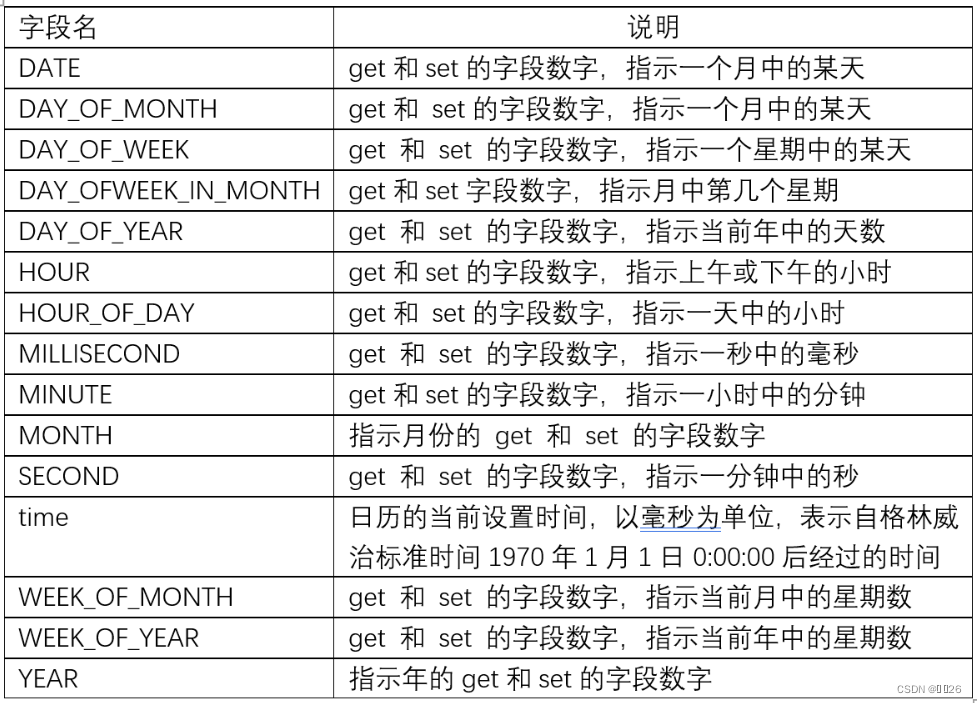Calendar類提供的常用字段及說明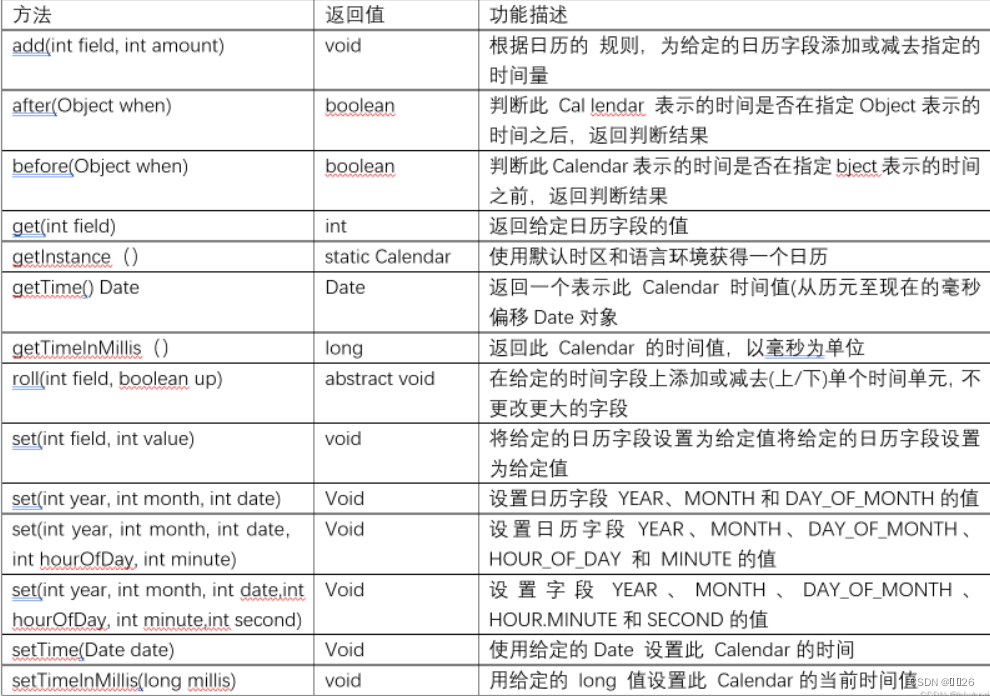``````package tt;    //類包
import java.text.SimpleDateFormat;//導入要用到的包
import java.util.Calendar;//導入要用到的包
import java.util.Date;//導入要用到的包
public class OlympicWinterGames {      //創建類public static void main(String[] args) {//主方法System.out.println("——————————冬奧會倒計時——————————\n");//輸入冬奧會倒計時Date date = new Date(); //實例化DateSimpleDateFormat simpleDateFormat = new SimpleDateFormat("yyyy-MM-dd"); //創建SimpleDateFormat對象，制定目標格式String today = simpleDateFormat.format(date);// 調用format方法，格式化時間，轉換為指定方法System.out.println("今天是" + today); //輸出當前日期long time1 = date.getTime(); //計算“自 1970 年 1 月 1 日 00:00:00 至當前時間所經過的毫秒數Calendar calendar = Calendar.getInstance(); //使用默認時區和語言環境獲得一個日歷calendarcalendar.set(2022, 2-1, 4); //設置日歷calendar中的 YEAR、MONTH 和 DAY_OF_MONTH 的值long time2 = calendar.getTimeInMillis(); //計算“自 1970 年 1 月 1 日 00:00:00 至 2022 年 2 月 2 日所經過的毫秒數long day = (time2 - time1)/(24 * 60 * 60 * 1000); //計算2022 年 2 月 2 日距離當前時間的天數System.out.println("距離2022年“北京-張家口”冬奧會還有 " + day + " 天！");//輸出距離2022年“北京-張家口”冬奧會還有多少天}
}``````